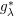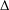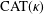Home

### Integral, integro-differential, and pseudodifferential operators (47Gxx)

• Article
• ##### ON THE BILINEAR SQUARE FOURIER MULTIPLIER OPERATORS ASSOCIATED WITH$g_{\unicode[STIX]{x1D706}}^{\ast }$ FUNCTION
• Nagoya Mathematical Journal, Volume 239

### Equations and inequalities involving nonlinear operators (47Jxx)

• Article
• #####$\unicode[STIX]{x1D6E5}$-CONVERGENCES OF WEIGHTED AVERAGED PROJECTIONS IN$\text{CAT}(\unicode[STIX]{x1D705})$ SPACES
• Journal of the Australian Mathematical Society, Volume 110, Issue 3